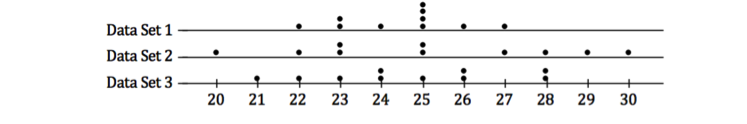# Descriptive Statistics

## Objective

Calculate the standard deviation and compare two symmetrical distributions based on the mean and standard deviation.

## Common Core Standards

### Core Standards

?

• HSS-ID.A.2 — Use statistics appropriate to the shape of the data distribution to compare center (median, mean) and spread (interquartile range, standard deviation) of two or more different data sets.

• HSS-ID.A.4 — Use the mean and standard deviation of a data set to fit it to a normal distribution and to estimate population percentages. Recognize that there are data sets for which such a procedure is not appropriate. Use calculators, spreadsheets, and tables to estimate areas under the normal curve.

?

• 7.SP.B.3

• 7.SP.B.4

## Criteria for Success

?

1. Describe standard deviation as a more concise way to measure variability because it is represented in the same units as the mean, not as the squared units (like the variance).
2. Describe how the formula for finding the standard deviation illustrates the spread of the data set.
3. Calculate by hand and then use the standard deviation function on a calculator to find the standard deviation of a symmetrical distribution.
4. Mark the standard deviations in a symmetrical distribution, identifying the units associated with the standard deviation.
5. Describe how the standard deviation of a data set indicates the spread of the data set.

## Tips for Teachers

?

This site, Bacon Bits, “Understanding Standard Deviation," succinctly explains standard deviation and includes the Excel formulas that are most applicable.

## Anchor Problems

?

### Problem 1

Here is the formula for standard deviation.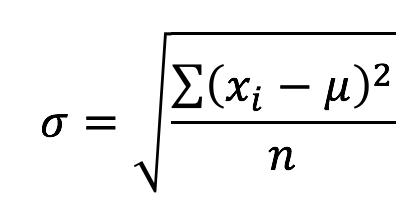Below are the definitions of the variables:
•    ${x }$ represents a data item.
•    ${x_{i}}$ represents EACH data item.
•    ${\mu }$ represents the mean.
•    ${\sum }$ represents the sum.
•    ${n }$ is the number of data items.
•    ${\sigma }$ is the standard deviation.

How would you describe the standard deviatio{n }using the definitio{n }of each variable?

### Problem 2

Compare the following three graphs. Explain why the standard deviations are different. Describe the shapes of the graphs to explain your reasoning.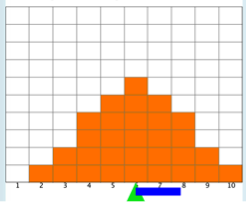Mean: 6
Standard Deviation: 1.9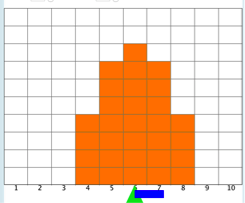Mean: 6
Standard Deviation: 1.2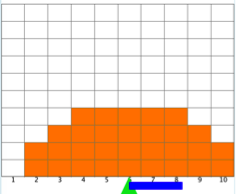Mean: 6
Standard Deviation: 2.3

## Problem Set

?

The following resources include problems and activities aligned to the objective of the lesson that can be used to create your own problem set.

• Write problems where the standard deviation is the same but the distribution and the mean are different. Explain how this can be.
• Write problems where the mean is the same, but the standard deviation is different. Explain how this can be.
• This applet is helpful to create problems where you can compare two different distributions and their standard deviations.